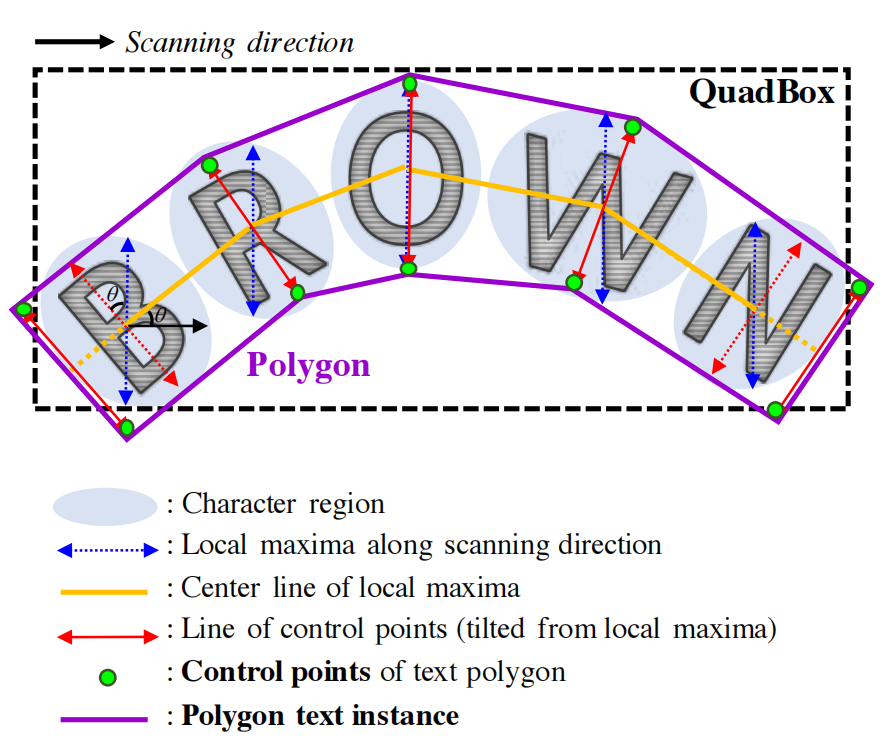# 文本检测的字符区域感知

## 1.引言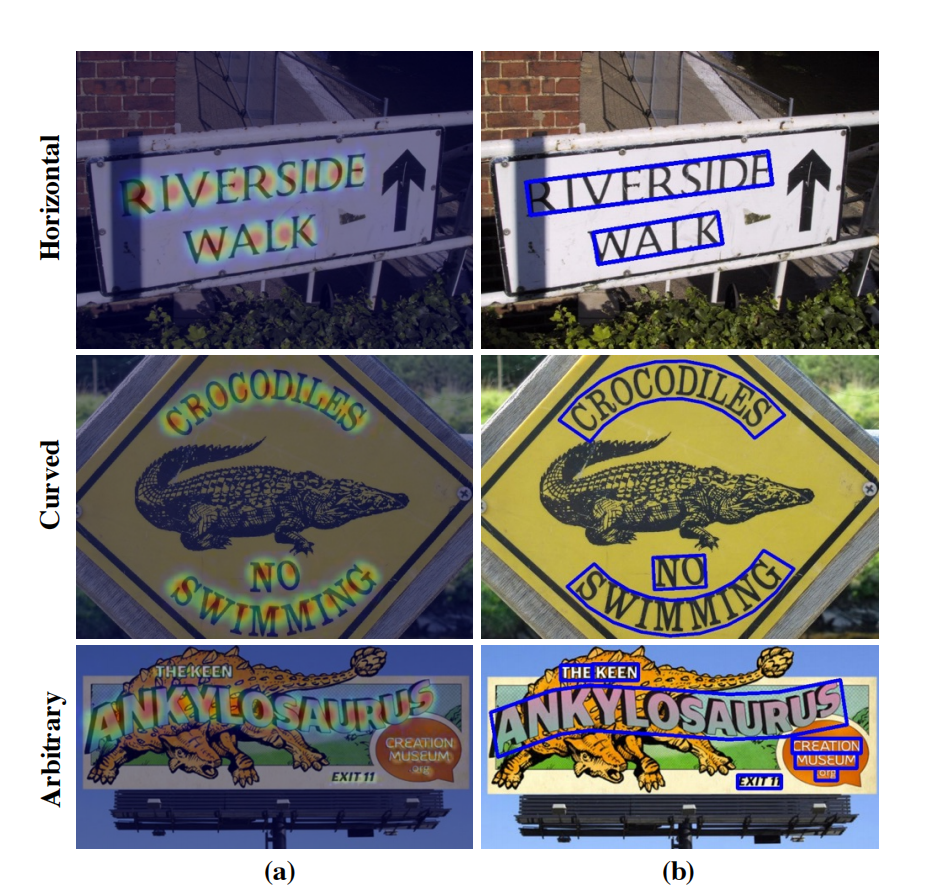## 3.方法

### 3.1结构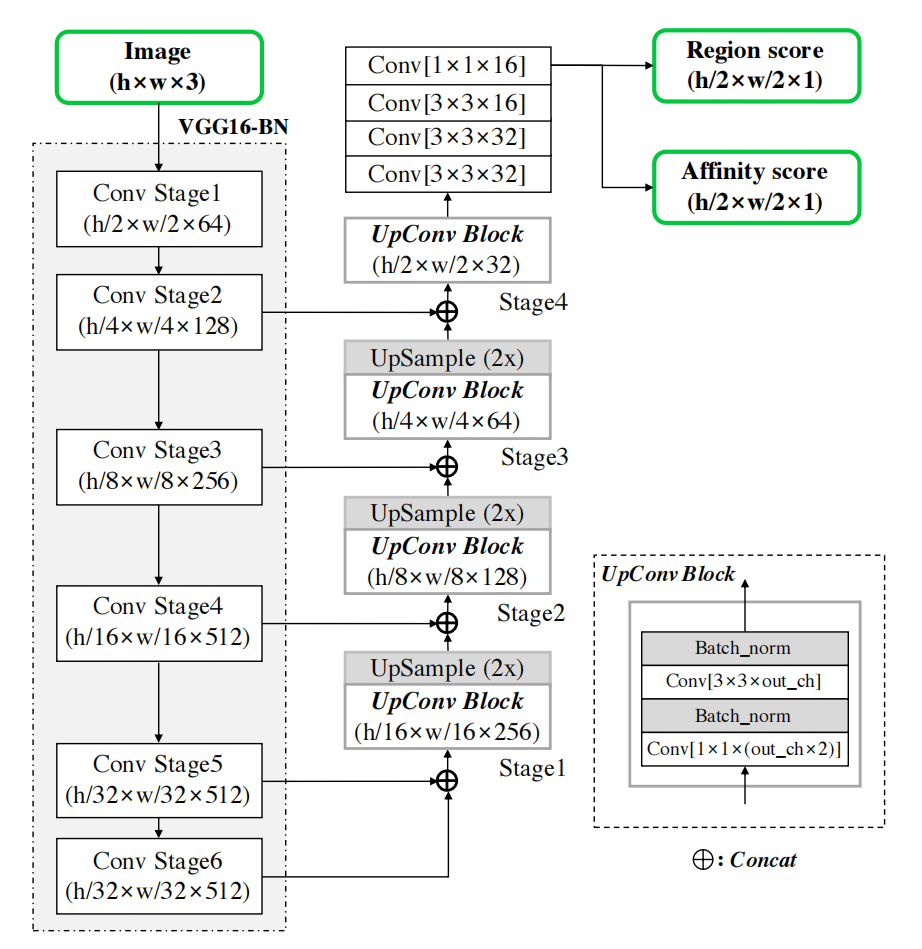### 3.2训练

#### 3.2.1真实标签生成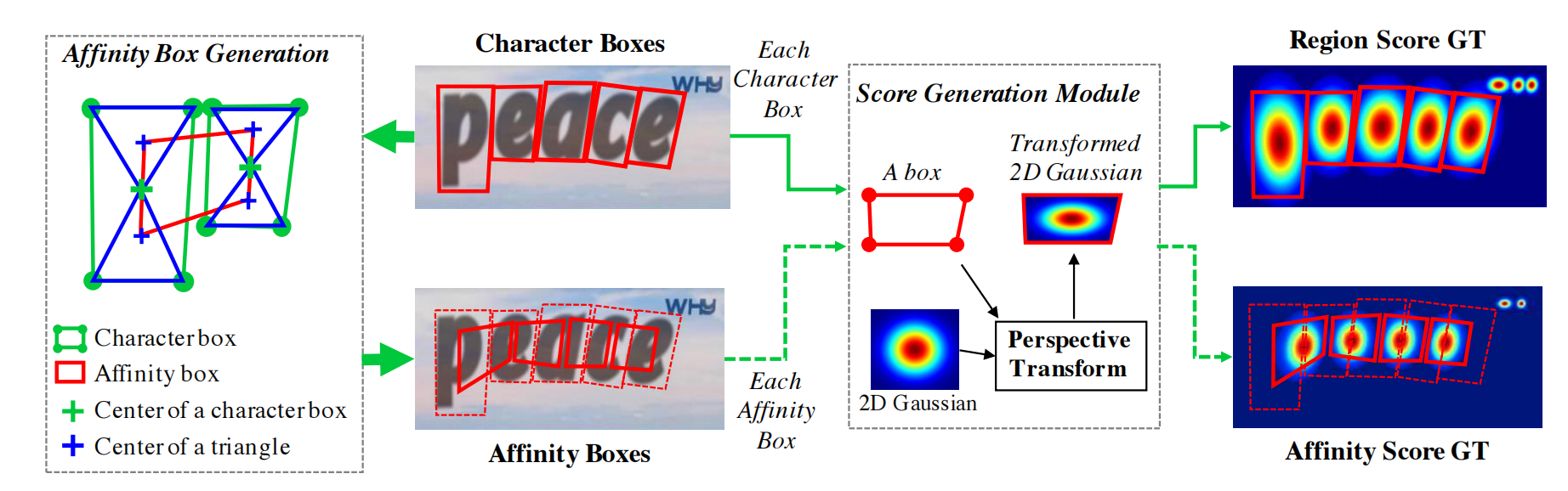#### 3.2.2弱监督学习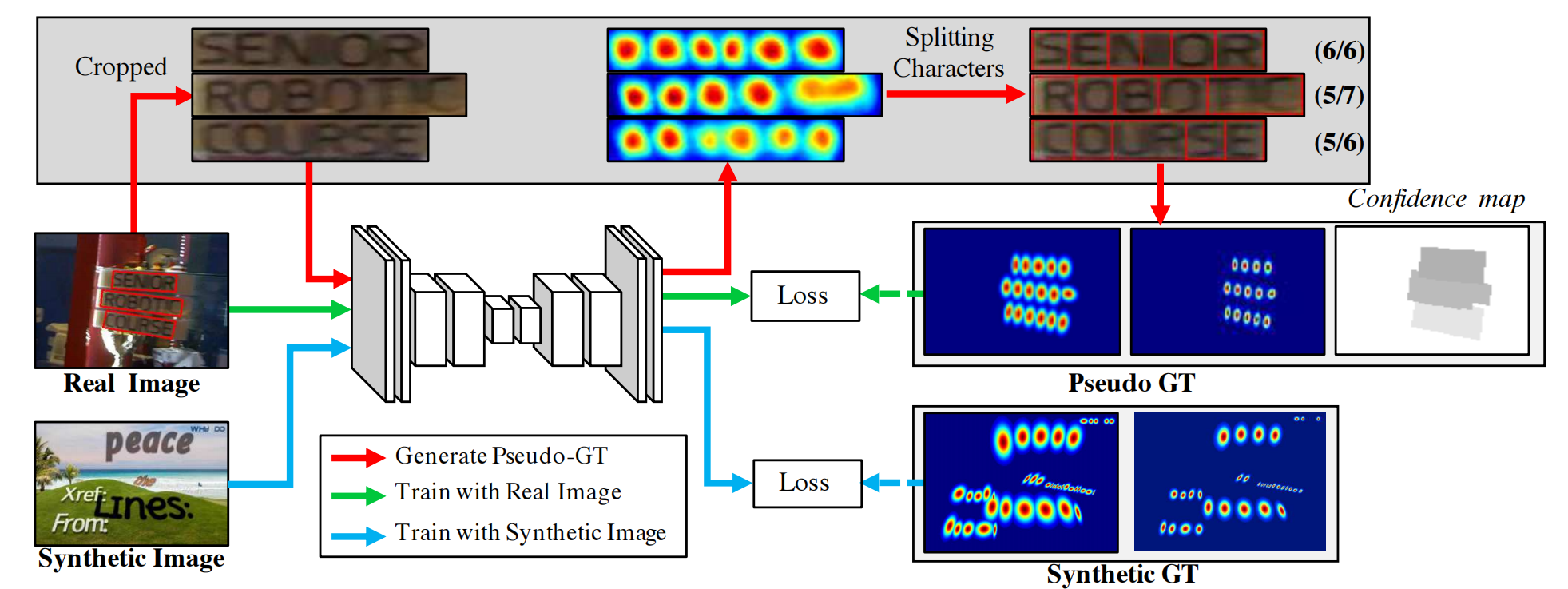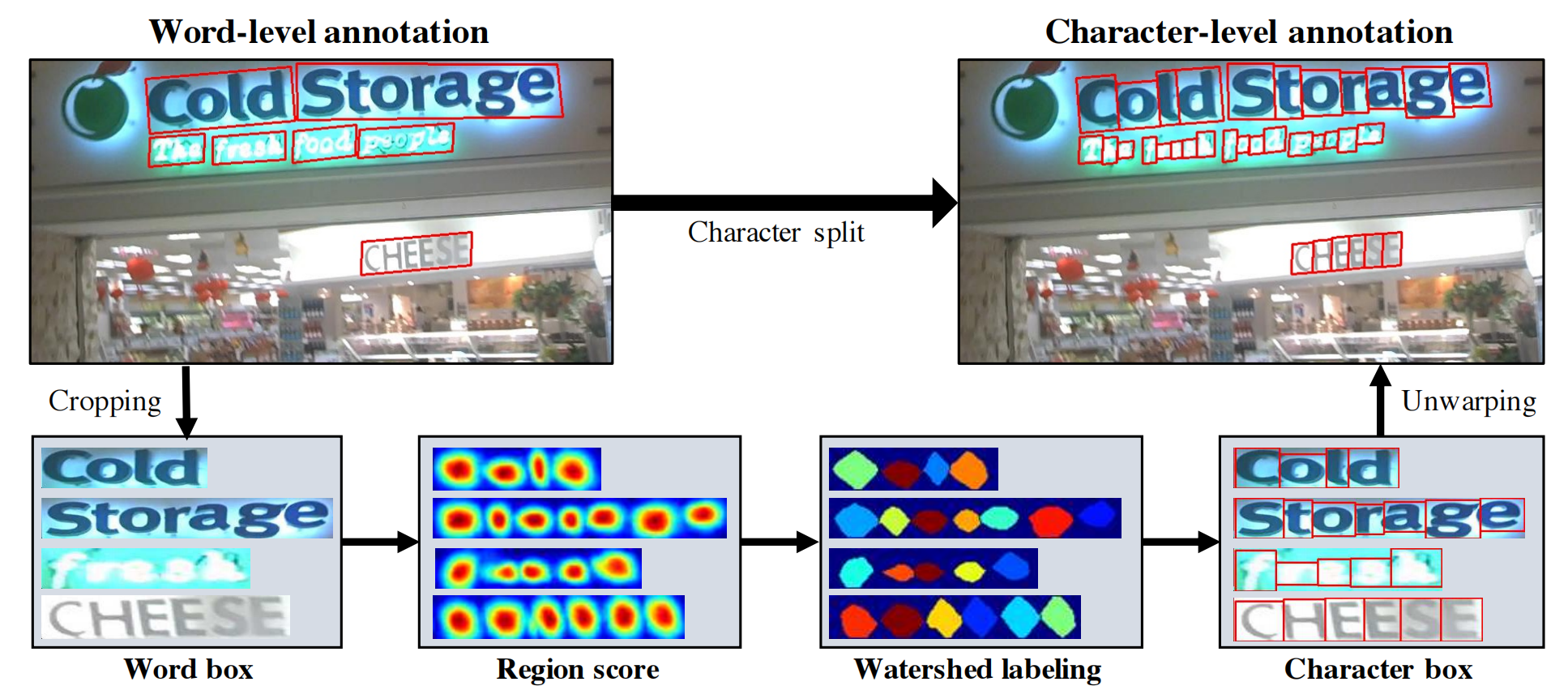• 然后将样本w的置信度得分sconf(w)计算为

$s_{conf}(w)=\frac{l(w)- min(l(w),|l(w)- l^{c}(w)|)}{l(w)} {,\qquad(1)}$

• 图像的像素置信度图Sc计算为

$S_{c}(p)= \begin{cases} s_{conf}(w) & \text{p\inR(w)}, \\[2ex] 1 & \text{otherwise}, \\ \end{cases} \qquad\qquad{(2)}$

• 其中p表示区域R（w）中的像素。目标L定义为

$L=\sum_{p}{S_{c}(p)\cdot(||S{_r}(p)-S{^*_r}(p)||{^2_2} +||S{_a}(p)-S{^*_a}(p)||{^2_2})} ,\quad(3)$

### 3.2推理